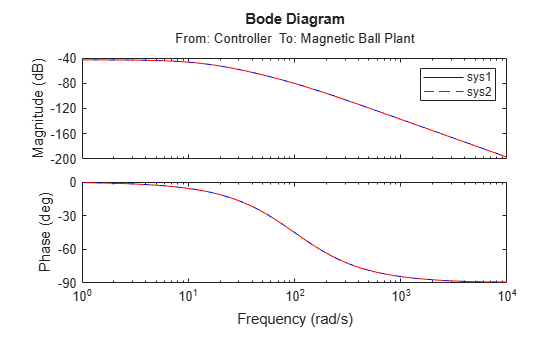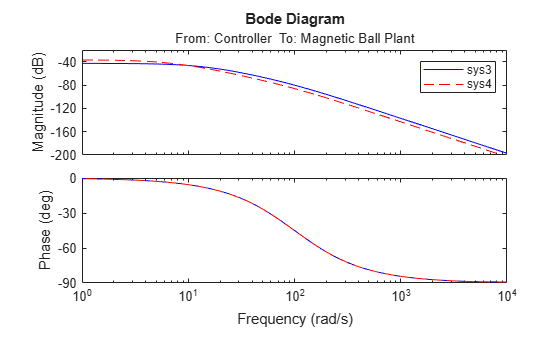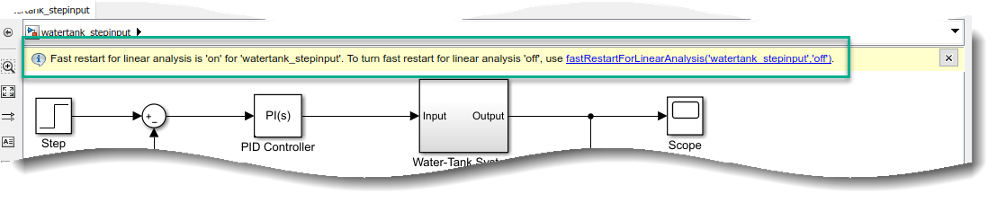fastRestartForLinearAnalysis

Fast restart for linear analysis

Description

example

fastRestartForLinearAnalysis(model,'on') prepares the model for single compilation workflows by turning fast restart for linear analysis 'on'. Once a compiling function is called, the model will remain compiled after the function is finished executing. Compiling functions can then be later called without any additional compilations. If the linear analysis points or block substitutions change with subsequent calls to compiling functions, the model is recompiled.

example

fastRestartForLinearAnalysis(model,'on',Name,Value) prepares the model for single compilation workflows with additional options specified by one or more Name,Value pair arguments.

example

fastRestartForLinearAnalysis(model,'off') turns fast restart for linear analysis off and restores the model parameters to their original value. Simulink® does not let you close the model while it is in a complied state. Use this syntax to turn fast restart for linear analysis off before closing the model.

You can also click the link that appears on the top of the compiled Simulink model to turn fastRestartForLinearAnalysis off. For more information, see Tips.

Examples

collapse all

Trim and linearize a closed-loop engine speed control model. Use fast restart for linear analysis to reduce the number of model compilations when looping over parameters.

Open the engine speed control model and get the analysis points for linearization. Doing so prevents recompilation between the first call to findop and linearize.

model = 'scdspeedctrl';
open_system(model)
io = getlinio(model);
fopt = findopOptions('DisplayReport','off');

Configure the PI controller to use the base workspace variables kp and ki.

block = [model,'/PID Controller'];
set_param(block,'P','kp');
set_param(block,'I','ki');

Create a grid of parameters to vary.

vp = 0.0005:0.0005:0.003;
vi = 0.0025:0.0005:0.005;
[KP,KI] = ndgrid(vp,vi);
N = numel(KP);
sz = size(KP);

Initialize the base workspace variables kp and ki.

kp = KP(1);
ki = KI(1);

Turn fastRestartForLinearAnalysis on and specify the analysis points using io.

fastRestartForLinearAnalysis(model,'on','AnalysisPoints',io)

Perform the linear analysis in a loop. When fast restart for linear analysis is on, calling findop sends the updated controller parameters to the model.

ops = operspec(model); % operating point specifications
for i = N:-1:1
kp = KP(i);
ki = KI(i);
op = findop(model,ops,fopt); % trim the model
[j,k] = ind2sub(sz,i);
sysFastRestartLoop(:,:,j,k) = linearize(model,io,op); % linearize the model
end

Turn off fastRestartForLinearAnalysis and close the model.

fastRestartForLinearAnalysis(model,'off')
bdclose(model)

When fast restart for linear analysis is on, calling compiling functions does not automatically apply changes to state initial conditions. Therefore, you must configure the initial state using an operating point object rather than using parameters or workspace variables.

Open the model and create linear analysis points.

mdl = 'magball';
open_system(mdl)
io(1) = linio([mdl '/Controller'],1,'input');
io(2) = linio([mdl '/Magnetic Ball Plant'],1,'openoutput');

Configure the ball height to use the hInitial workspace variable as the initial condition.

set_param([mdl '/Magnetic Ball Plant/height'],'InitialCondition','hInitial')

Turn on fast restart for linear analysis.

fastRestartForLinearAnalysis(mdl,'on')

Linearize the model using different initial height values.

hInitial = 0.05;
sys1 = linearize(mdl,io);
hInitial = 0.1;
sys2 = linearize(mdl,io);

The frequency responses of the linearized models are the same. Therefore, the initial condition does not update when hInitial changes.

bode(sys1,'b',sys2,'r--')
legend('sys1','sys2')To vary the initial state when fast restart is enabled, you must modify an operating point object instead. Create an operating point based on the model initial conditions.

op = operpoint(mdl);

Modify the operating point for different initial conditions and linearize the model.

op.States(5).x = 0.05;
sys3 = linearize(mdl,io,op);
op.States(5).x = 0.1;
sys4 = linearize(mdl,io,op);

The frequency responses of the linearized models are different. Therefore, the initial condition changes when the operating point object changes.

bode(sys3,'b',sys4,'r--')
legend('sys3','sys4')Turn off fast restart for linear analysis and close the model.

fastRestartForLinearAnalysis(mdl,'off')
bdclose(mdl)

Input Arguments

collapse all

Simulink model name, specified as a character vector, string, slTuner object, or slLinearizer object. The model must be in the current working folder or on the MATLAB® path.

Name-Value Arguments

Specify optional comma-separated pairs of Name,Value arguments. Name is the argument name and Value is the corresponding value. Name must appear inside quotes. You can specify several name and value pair arguments in any order as Name1,Value1,...,NameN,ValueN.

Example: ...,'UseBusSignalLabels','on'

Analysis point set that contains inputs, outputs, and openings, specified as the comma-separated pair consisting of AnalysisPoints and a linearization I/O object or a vector of linearization I/O objects. To create AnalysisPoints:

• Define the inputs, outputs, and openings using linio.

• If the inputs, outputs, and openings are specified in the Simulink model, extract these points from the model using getlinio.

Each linearization I/O object in AnalysisPoints must correspond to the Simulink model model or some normal mode model reference in the model hierarchy.

If the linear analysis points change with subsequent calls to compiling functions, the model is recompiled.

For more information on specifying linearization inputs, outputs, and openings, see Specify Portion of Model to Linearize.

Substitute linearizations for blocks and model subsystems, specified as the comma-separated pair consisting of BlockSubstitutions and one of the following:

• A structure array

• A string array

• A character vector

• A cell array of character vectors

Use BlockSubstitutions to specify a custom linearization for a block or subsystem. For example, you can specify linearizations for blocks that do not have analytic linearizations, such as blocks with discontinuities or triggered subsystems.

If the block substitutions change with subsequent calls to compiling functions, the model is recompiled.

Flag indicating whether to use bus signal channel numbers or names to label the I/Os in the linearized model, specified as the comma-separated pair consisting of UseBusSignalLabels and one of the following:

• 'off' — Use bus signal channel numbers to label I/Os on bus signals in the linearized model.

• 'on' — Use bus signal names to label I/Os on bus signals in the linearized model. Bus signal names appear in the results when the I/O points are at the output of the following blocks:

• Root-level inport block containing a bus object

• Bus creator block

• Subsystem block whose source traces back to the output of a bus creator block

• Subsystem block whose source traces back to a root-level inport by passing through only virtual or nonvirtual subsystem boundaries

Tips

• Simulink does not let you close the model while it is in a compiled state. Turn fast restart for linear analysis off to close the model. You can turn off fastRestartForLinearAnalysis in one of the following ways.

• Use the syntax fastRestartForLinearAnalysis(model,'off').

• Click the link that appears on the top of the Simulink model.• When fast restart for linear analysis is on, calling compiling functions does not automatically apply changes to state initial conditions. Therefore, you must configure the initial state using an operating point object rather than using parameters or workspace variables. For more information, see Linearize Model Using Multiple State Initial Conditions.